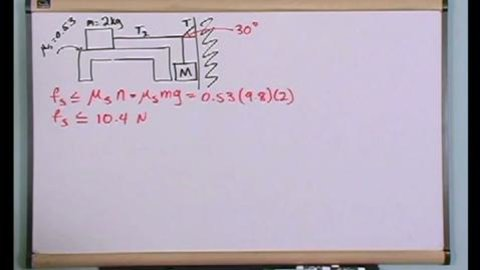# Newton’s Laws Of Motion Part of the Series: Physics Tutor Series: Learning By Example

##### TMW MediaShow More

## Related videos

Newton’s Laws Of Motion With Friction
Physics is frequently one of the hardest subjects for students to tackle because it is a combination of two of the toughest subjects for most students: Math and Word Problems. What sets this series apart from other physics teaching tools is that the concepts are taught entirely through step-by step…
Using Newton's Laws: 1-D motion
Investigate Newton's second law, which relates force, mass, and acceleration. Focus on gravity, which results in a force, called weight, that's proportional to an object's mass. Then take a ride in an elevator to see how your measured weight changes due to acceleration during ascent and descent.
Gravitation and Kepler’s Laws Of Motion
Physics is frequently one of the hardest subjects for students to tackle because it is a combination of two of the toughest subjects for most students: Math and Word Problems. What sets this series apart from other physics teaching tools is that the concepts are taught entirely through step-by step…
Newton's Laws in 2 and 3 Dimensions
Consider Newton's laws in cases of two and three dimensions. For example, how fast does a rollercoaster have to travel at the top of a loop to keep passengers from falling out? Is there a force pushing passengers up as the coaster reaches the top of its arc? The answer…
Reversibility and the Laws of Physics
Isaac Newton's laws of physics are fully reversible; particles can move forward or backward in time without any inconsistency. But this is not our experience in the world, where the arrow of time is fundamentally connected to irreversible processes and the increase in entropy.
Time’s Arrow
Embark on the quest that will occupy the rest of the course: Why is there an arrow of time? Explore how memory and aging orient us in time. Then look at irreversible processes, such as an egg breaking or ice melting. These capture the essence of the one-way direction of…
Electric Charge & Coulomb's Law Part 1
This program covers the important topic of Electric Charge and Coulomb's Law in Physics. We begin by discussing what electric charge is and what coulomb's law is. We take time to introduce the units to the student so that there is no confusion as to what the problem is asking.…
Electric Charge & Coulomb's Law Part 2
This program covers the important topic of Electric Charge and Coulomb's Law in Physics. We begin by discussing what electric charge is and what coulomb's law is. We take time to introduce the units to the student so that there is no confusion as to what the problem is asking.…
Gauss' Law Part 1
This program covers the important topic of Gauss' Law in Physics. We begin by discussing what Gauss' Law is. Next, we introduce the theory behind Gauss' Law and use diagrams to describe why it is important. The entire lesson is taught by working example problems beginning with the easier ones…
Gauss' Law Part 2
This program covers the important topic of Gauss' Law in Physics. We begin by discussing what Gauss' Law is. Next, we introduce the theory behind Gauss' Law and use diagrams to describe why it is important. The entire lesson is taught by working example problems beginning with the easier ones…
Gauss's Law Part 3
This program covers the important topic of Gauss' Law in Physics. We begin by discussing what Gauss' Law is. Next, we introduce the theory behind Gauss' Law and use diagrams to describe why it is important. The entire lesson is taught by working example problems beginning with the easier ones…
Gauss' Law Cylindrical Symmetry
This program covers the important topic of Gauss' Law with Cylindrical Symmetry in Physics. We begin by discussing when Cylindrical Symmetry can be applied to Gauss' Law problems to make them easier to solve. The entire lesson is taught by working example problems beginning with the easier ones and gradually…